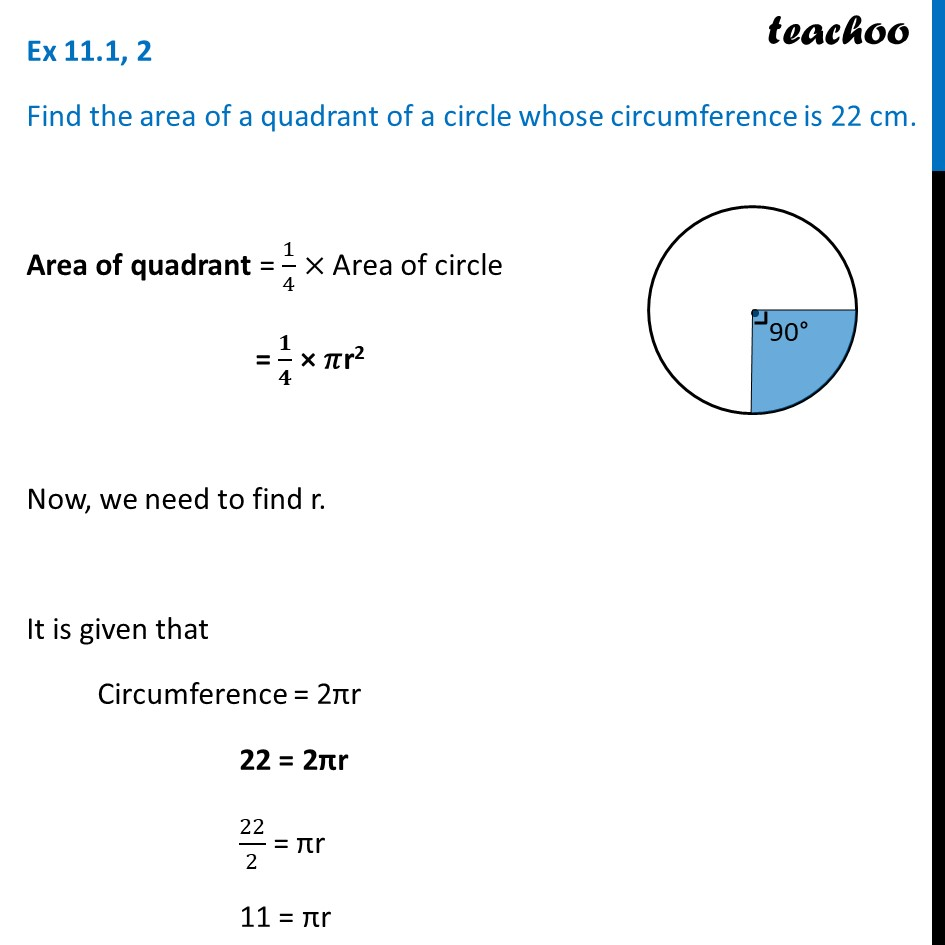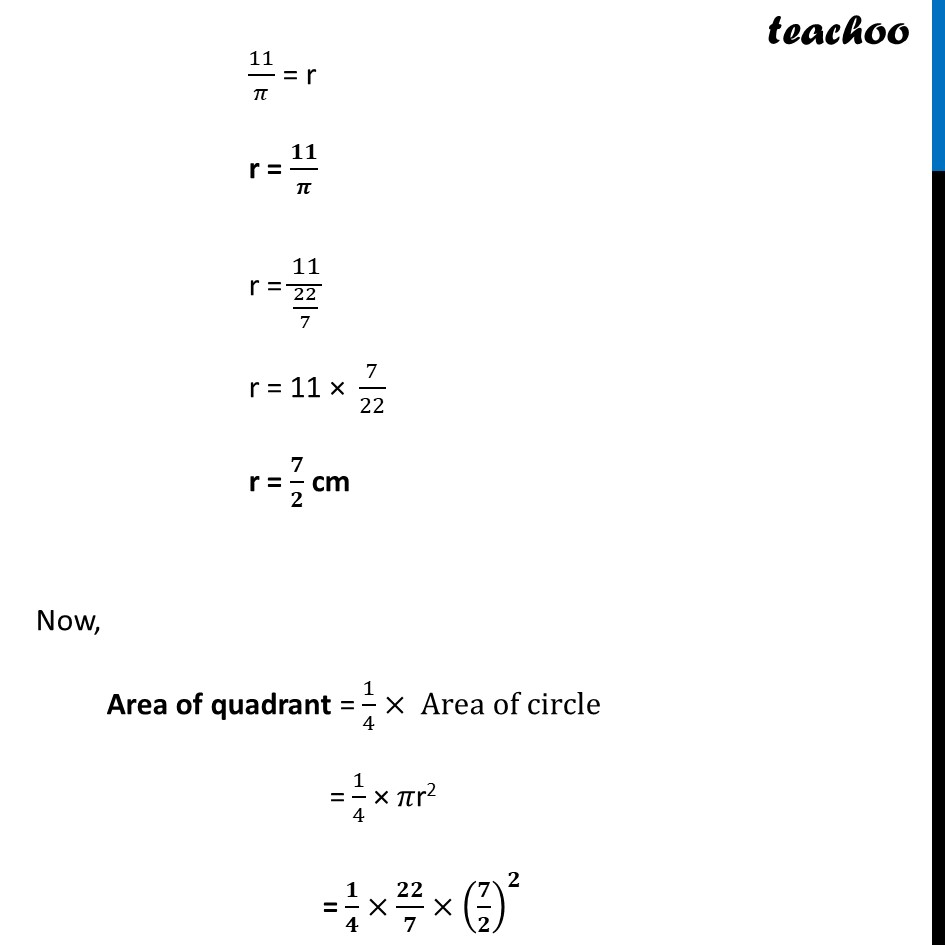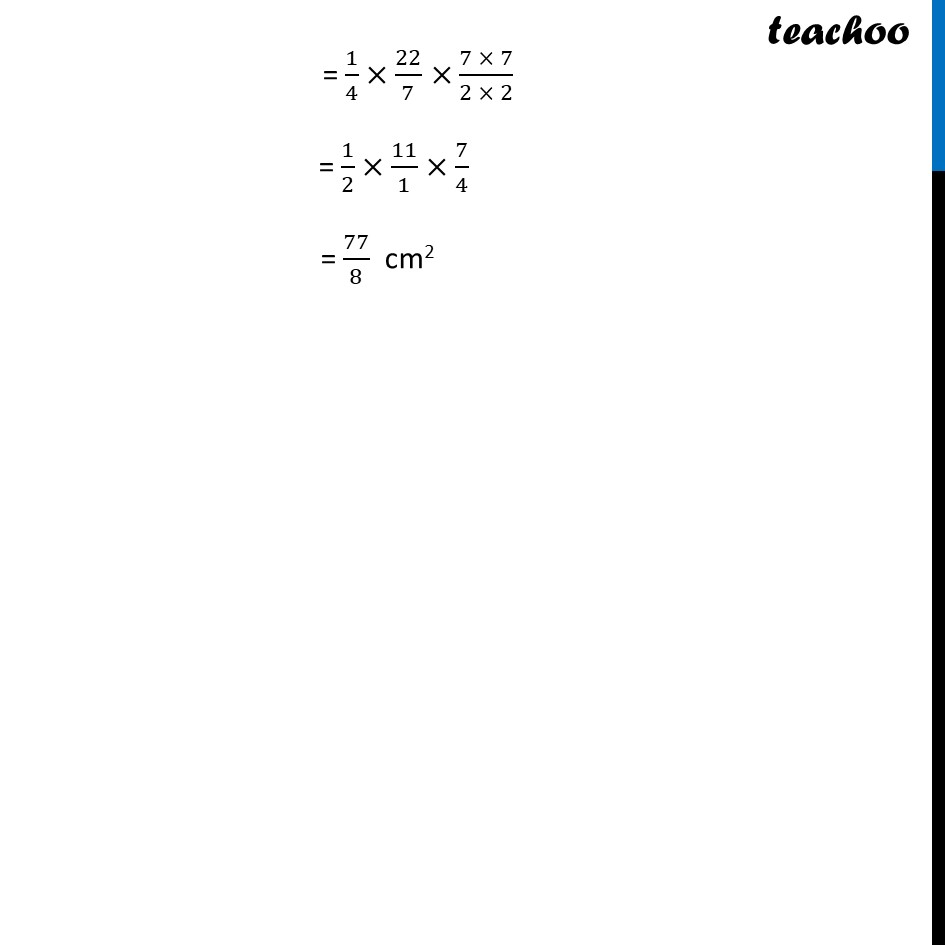Area of sector of circle

Chapter 11 Class 10 Areas related to Circles
Concept wiseLearn in your speed, with individual attention - Teachoo Maths 1-on-1 Class

### Transcript

Ex 11.1, 2 Find the area of a quadrant of a circle whose circumference is 22 cm. Area of quadrant = 1/4× Area of circle = 𝟏/𝟒 × 𝜋r2 Now, we need to find r. It is given that Circumference = 2πr 22 = 2πr 22/2 = πr 11 = πr 11/𝜋 = r r = 𝟏𝟏/𝝅 r = 11/(22/7) r = 11 × 7/22 r = 𝟕/𝟐 cm Now, Area of quadrant = 1/4× Area of circle = 1/4 × 𝜋r2 = 𝟏/𝟒×𝟐𝟐/𝟕×(𝟕/𝟐)^𝟐 = 1/4×22/7×(7 × 7)/(2 × 2) = 1/2×11/1×7/4 = 77/8 cm2